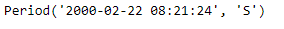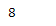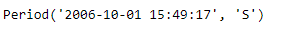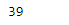Python | Pandas Period.week

• Last Updated : 06 Jan, 2019

Python is a great language for doing data analysis, primarily because of the fantastic ecosystem of data-centric python packages. Pandas is one of those packages and makes importing and analyzing data much easier.

Pandas Period.week attribute returns an integer value which represents the week number in which the given period lies.

Syntax : Period.week

Parameters : None

Return : week of the year

Example #1: Use Period.week attribute to find out the week in which the given period object would lie.

 # importing pandas as pdimport pandas as pd  # Create the Period objectprd = pd.Period(freq ='S', year = 2000, month = 2, day = 22,                         hour = 8, minute = 21, second = 24)  # Print the Period objectprint(prd)

Output :Now we will use the Period.week attribute to find the week in which prd object lies.

 # return the week valueprd.week

Output :As we can see in the output, the Period.week attribute has returned 8 indicating the following period object lies in the 8th week of the given year.

Example #2: Use Period.week attribute to find out the week in which the given period object would lie.

 # importing pandas as pdimport pandas as pd  # Create the Period objectprd = pd.Period(freq ='S', year = 2006, month = 10,               hour = 15, minute = 49, second = 17)  # Print the objectprint(prd)

Output :Now we will use the Period.week attribute to find the week in which prd object lies.

 # return the week valueprd.week

Output :As we can see in the output, the Period.week attribute has returned 39 indicating the following period object lies in the 39th week of the given year.

My Personal Notes arrow_drop_up